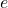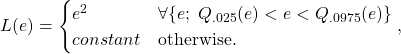# Outliers and Loss Functions

Contents

## A few words about outliers

In statistics, outliers are as thorny topic as it gets. Is it legitimate to treat the observations seen during global financial crisis as outliers? or are those simply a feature of the system, and as such are integral part of a very fat tail distribution?

I recently read a paper where the author chose to remove forecasts which produced enormous errors:

(some) models produce erratic forecasts on some occasions, and these have an undue impact on the reported mean squared prediction errors.. To mitigate the impacts of such forecasts, (..) if a forecast is more than five standard deviations away from the mean, it is replaced by the mean.

At first glance this looks like: “Oh, that’s rich, so when you don’t like the backtest results, you shave-off the worst outcomes not to taint the accuracy”. However, come to think about it, it is not as unacceptable as it looks.

What is going on when we remove outliers? We can cast this question in terms of loss functions.

## Loss functions quick review

### Square loss

By far the most common loss function is the mean squared error. If there is no difference between the actual observation and your forecast there is no loss (loss=0). The loss increase exponentially as you miss your target to the left or to the right, symmetrically. So the price you pay for missing the target by two units is 4, if you miss by 4 units the price is then 16.We like this function because it is mathematically convenient to work with, and because of the intuitive notion that large errors should be penalized much more heavily.

### Absolute loss

Another increasingly popular loss function is the absolute loss function. This time larger errors are associated with larger penalties, but not much larger; they increase only linearly, as oppose to exponentially as before.The absolute loss function used to be much less common given that it is not differentiable at zero. However, it is now quite standard to use it because of a widespread implementation of numerical solutions, now standard in all statistical software. And of course the fact that LASSO uses this loss function does not hurt.

### Epsilon-insensitive loss

This loss function is not very intuitive. Small errors are not penalized at all. The primary use of this function is for the Support Vector Machine algorithm I think.## Outliers and loss functions coming together

Essentially, when you remove your funny looking forecasts it is not exactly like ignoring them. There is still a penalty, it is simply an average penalty, but it is not zero. To be exact the loss function after removing outliers (say 5% extremes, so 2.5% each side) looks like that:whereis the loss function, andis the error (difference between prediction and target). I mean to say that there is still a cost, which is not zero. This is on the math side, but we can argue further. Funny looking forecasts are often overruled in practice, so also on that ground we need not introduce those large errors in our backtest.

You don’t have to go with a built-in loss function. It is quite simple to define own loss (or sometimes called cost) function. For example, you can introduce asymmetry, or you can cap the penalty of large errors:

### Truncated loss

You don’t have to set the loss of a large error at the average, you can decide on a fixed cost, which would mean formally that:which would look like:So that you have a constant penalty which is large, rather than the average penalty.

## Practical example

Here I provide some code to start you off if you want to use your own loss function. We would also be able to see what is the impact of using epsilon-insensitive loss; this would help you get some intuition for the impact of changing the objective function from the usual squared loss.

Here is the result of the fitted values from both the linear model which uses the familiar square loss function and the fit from optimizing using epsilon insensitive loss function.The epsilon insensitive loss function cares less about what is happening in the center and tracks more closely the extremes. By the definition the center in this simple model is x=0 with y=2. The epsilon insensitive loss function has a better match for y-points which are far from the center, compared with the square loss function, which is expected. This is on the expense of errors around the center where the green line tracks more closely. The overall RMSE is not very different, just different preferences.

## Summary

(2) You can create your own loss function based on own preferences, risk aversion (maybe try f = abs(()^3)?), asymmetries, kinks or what not. The code can start you off, optimized here using what is dubbed Epsilon Insensitive Loss; you can adjust it to your liking.1.Dir says: